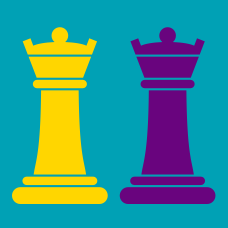Logic

Chess Tactical: Level 4 Challenges

In the position below, how can White give the fastest checkmate? (Assume both players are moving optimally.)

The target square of the first move can be represented as a coordinate $(x,y)$, where the bottom left corner is at $(0,0)$. Input the product of $x$ and $y$ as your answer.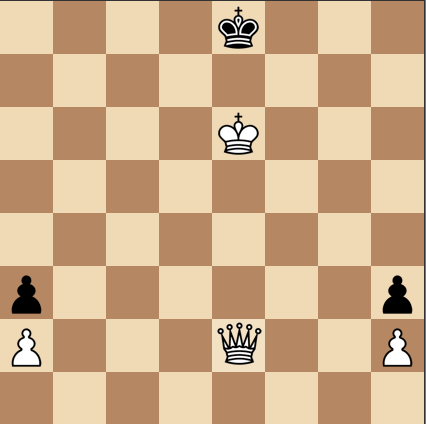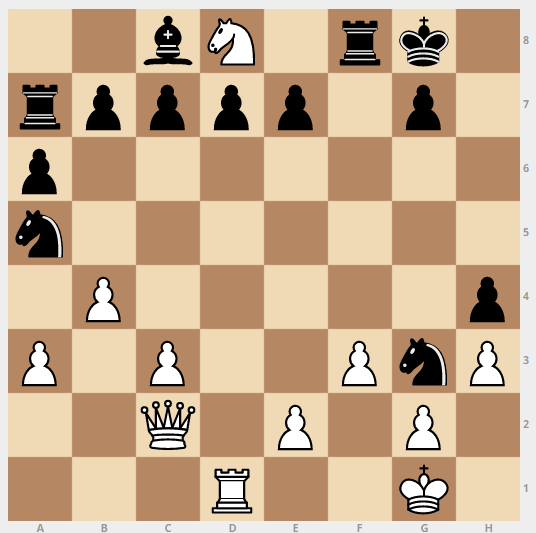In our extraordinary game,

• Black has castled in the last move,
• In one of the 3 previous moves, the white knight from g1 was captured on its initial square.

Which piece captured the knight?

This problem is the second of the set Retrograde Chess.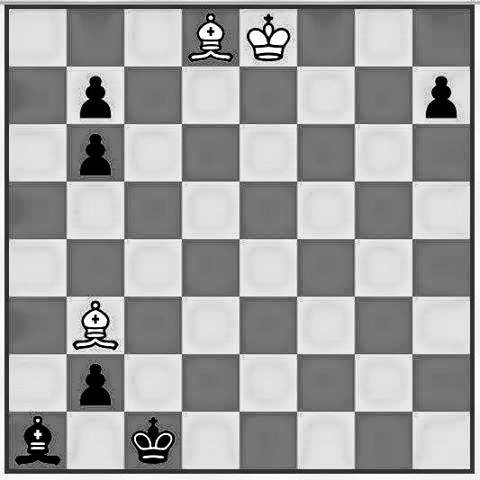It's White turn to move, find the minimum number of moves for White to move to win the game.

Note that both players played optimally and that Black moves down (pawn) and that Black intends to stay in the game for as long as possible.

Designed by Fikri Prayoga.

In the following position, the color of each piece is unknown (each piece could either be white or black), but it is known that the position arose from a series of legal moves, starting at the usual starting position.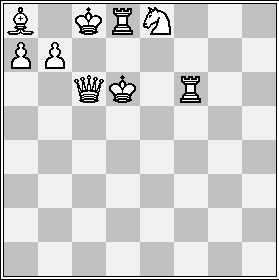Before the last move was made, Black had $a$ pieces worth $b$ points (on the scale Queen = 9, Rook = 5, Bishop = Knight = 3, Pawn = 1, King = 0). What is the product of $a$ and $b$?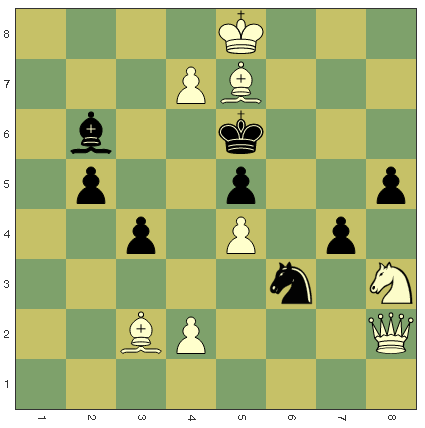Consider the positional set up of a game. It is white's turn to move. White can checkmate black in just two moves, can you find the first move to force a checkmate?

If the first move can be written as $(x_1,y_1) , (x_2,y_2)$, where $x_1,y_1$ are the coordinates (according to how it is marked above) of the moved piece's initial position and $x_2,y_2$ are the coordinates of the position of the piece after the move, evaluate $(x_1+y_1) \times (x_2+y_2)$

×